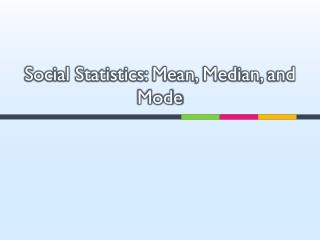DownloadDownload PresentationSocial Statistics: Mean, Median, and Mode

Social Statistics: Mean, Median, and Mode

Télécharger la présentationSocial Statistics: Mean, Median, and Mode

- - - - - - - - - - - - - - - - - - - - - - - - - - - E N D - - - - - - - - - - - - - - - - - - - - - - - - - - -
Presentation Transcript

1. Social Statistics: Mean, Median, and Mode

2. This week • Mean • Median • Mode S519

3. How do we decide which is “best”? • The overall goal of central tendency is to find the single score that is most representative for the distribution. S519

4. Measures of Central Tendency • Mean: Arithmetic average • sum of scores divided by number of scores • most frequently used b/c it uses all scores in the set • Median: “Middle” score, when scores are in order • corresponds to the 50th percentile • appropriate for skewed/open-ended distributions, and • distributions with undetermined scores • Mode: Most frequently occurring (popular) score • appropriate for nominal data S519

5. Mean (x bar): the mean : sum of the data : number of the data S519

6. Mean • The sample mean is the measure of central tendency which can approximate the population mean • The mean is very sensitive to extreme scores • It can put the mean in some extreme direction • Make it less representative • Less useful as a measure of central tendency S519

7. Calculate mean • The mean or average number of shoppers in each store? • Using Excel to do that • use your own formula • use AVERAGE function S519

8. Median • It is defined as the midpoint in a set of scores • 50% of the scores fall above and one half fall below. S519

9. Calculate median • Odd number of data • Rank them • Median=middle one • Example: 10, 9, 8, 7, 5 (median=8) • Even number of data • Rank them • Median= sum of two middle data/2 • Example: 10, 9, 8, 7, 6, 5 (median=(8+7)/2=7.5) S519

10. Median • The median is insensitive to extreme cases, where the mean is not. • To measure the central tendency: • Have some extreme data, using median • No extreme data, using mean • Example: 14, 3, 2, 1, (mean=5, median=2.5) • Which represents better the central tendency? S519

11. Median in Excel • Calculate the median of income level S519

12. Mode • The mode is the value that occurs most frequently. • Calculate the frequency of all the values in a distribution • The value that occurs most often is the mode S519

13. Calculate mode • 185 students: Mode = american student S519

14. When to use what • Mean: • No extreme scores and are not categorical • Median • Extreme scores and you do not want to distort the average • Mode • Data are categorical in nature and values can only fit into one class • E.g. hair color, political affiliation, religion S519

15. Descriptive Statistics in Excel • Take Figure2.9 (S-p57), input these figures to Excel • Data  data analysis  data analysis box  choose Descriptive Statistics  tick “labels in first row”  output range=c1  tick “summary statistics”  click “OK” S519

16. Exercise 1 (S-p62) • Calculate mean, median and mode for the following data: S519

17. Exercise 2 (S-p62) • Writing a sale report to your boss according to the figures of things sold today: S519

18. Exercise 4 (S-p63) • Calculate the average sale S519

19. Exercise 5 (S-p63) • Patient record • Mean and median, which is better for what? S519